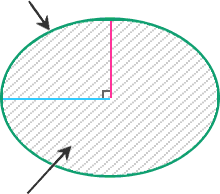# Ellipse Perimeter/Circumference Calculator

This calculator is used for quickly finding the perimeter (circumference) of an ellipse. And even more. You can also use it to find an ellipse area. Just enter a semimajor axis length. Then a semiminor axis length. Tap or click the Calculate button. Get the result. The result will also be shown in the picture.
The perimeter of an ellipse is very hard to find. This calculator uses Ramanujan's second approximation. It is one of the most accurate of its kind.

a(semimajor axis):

b(semiminor axis):

P(perimeter):

A(area):

π(pi):

## Solution

P ≈ π(a+b)(1+
3h10+√(4-3h)
)
where h = ((a-b)/(a+b))2 =
A = πab =a

b

P

A

Note: Most ellipse perimeter / circumference calculators find only approximate results. Our calculator gives you the most accurate one. The result can be too big or too small. In this case, it will be shown in scientific notation.

It's Interesting

## Ellipse formulas used by this calculator:

### For the Perimeter (Circumference)

P ≈ π(a+b)(1+
3h10+√(4-3h)
)
where a is a semimajor axis of the ellipse, b is a semiminor axis of the ellipse and h = ((a-b)/(a+b))2
Note: This formula is called "Ramanujan's second approximation". Though it degrades for large b/a ratios, it is one of the most accurate approximations. That is why, it is used to find the perimeter (circumference) in our calculator.

### For the Area

A = πab
where a is a semimajor axis of the ellipse, b is a semiminor axis of the ellipse, and π (pi number) is a mathematical constant ≈ 3.141592653589793